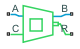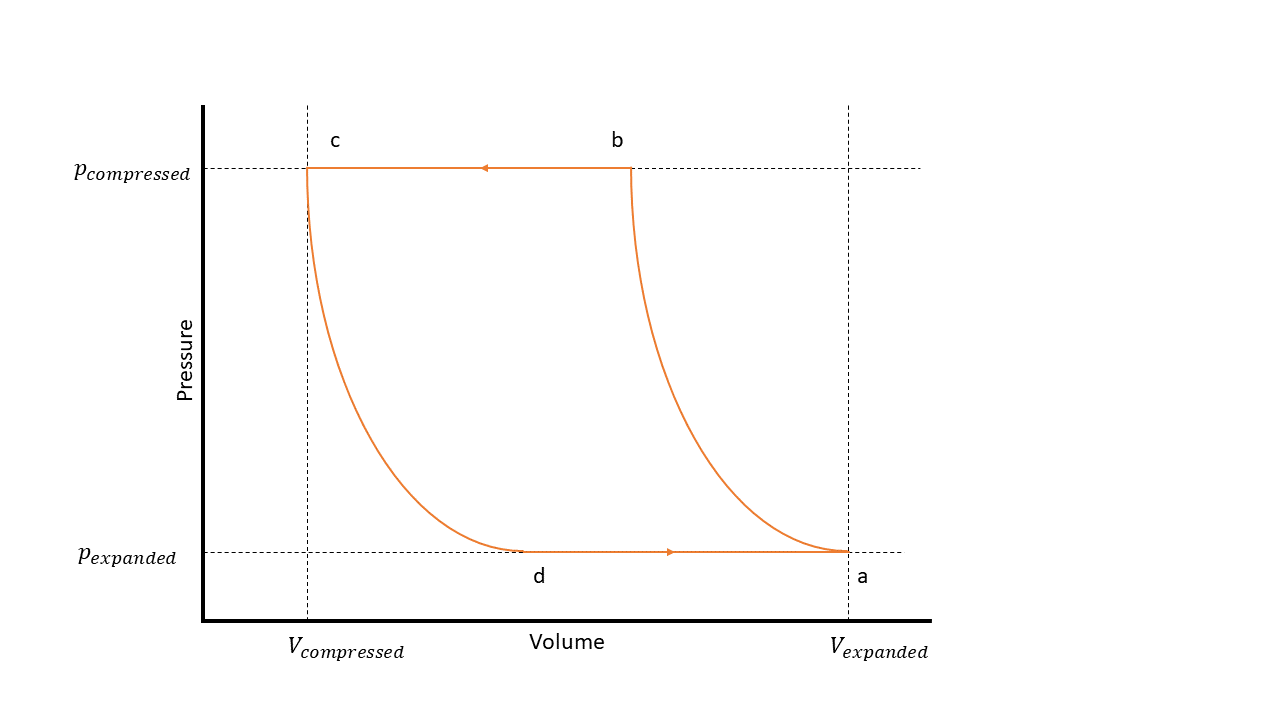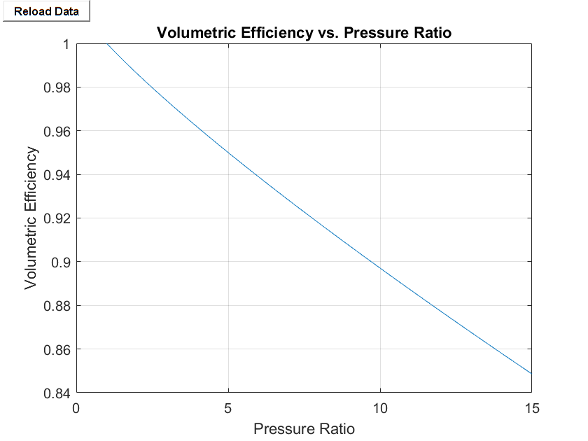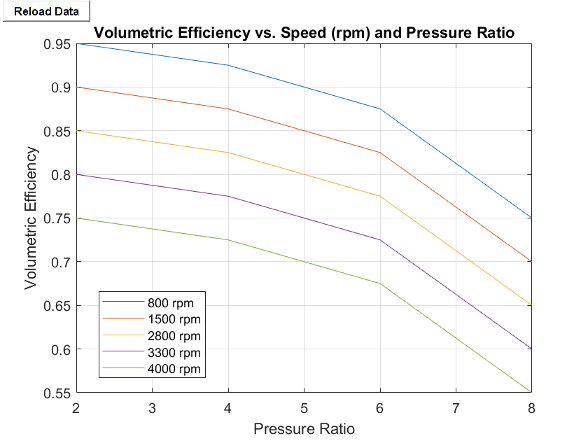# Positive-Displacement Compressor (2P)

Two-phase fluid positive-displacement compressor in a thermodynamic cycle

• Library:
• Simscape / Fluids / Two-Phase Fluid / Fluid Machines

•## Description

The Positive-Displacement Compressor (2P) block represents a positive-displacement compressor, such as reciprocating piston, rotary screw, rotary vane, and scroll, in a two-phase fluid network. You can control how the block parameterizes a positive displacement compressor by setting Parameterization to `Analytical` or `Tabulated`. Port R and port C are mechanical rotational conserving ports associated with the compressor shaft and casing, respectively. When there is positive rotation at port R with respect to port C, two-phase fluid flows from port A to port B. The block may not be accurate for reversed flow.

The block treats the positive displacement process as polytropic such that pvn is constant, where p is pressure, v is specific volume, and n is the Polytropic exponent parameter. You can use the block to model isentropic processes by setting n equal to the isentropic exponent, k. The figure shows the steps of a positive-displacement compressor on a P-V diagram, which has these states:

• a — The compressor cylinder is full at inlet pressure.

• b — The pressure inside the compressor exceeds that of the outlet, which results in fluid discharge.

• c — The compressor reaches the top of the piston stroke, and only the clearance volume remains in the cylinder.

• d — The pressure inside the cylinder drops below the inlet pressure, which results in fluid intake.### Efficiency

When you set Volumetric efficiency parameterization to `Analytical`, the block calculates the volumetric efficiency as

`${\eta }_{V}=1+C-C{\left(\frac{{p}_{out}}{{p}_{in}}\right)}^{1}{n}},$`

where the block implements the polytropic pressure-volume relationship. The block calculates the clearance volume fraction, C, as

`$C=\frac{1-{\eta }_{{V}_{nominal}}}{{p}_{ratio}{}^{1/n}-1},$`

where ηVnominal is the Nominal volumetric efficiency parameter, and pratio is the ratio of saturation pressures that correspond to Nominal condensing temperature and Nominal evaporating temperature.

When you set Volumetric efficiency parameterization to

`Tabulated`, the block interpolates the values of the Volumetric efficiency table, eta(w,pr) parameter as a function of the shaft speed and the pressure ratio.

### Continuity Equations

The block calculates the mass flow rate as

`$\stackrel{˙}{m}={\eta }_{V}\omega \frac{{V}_{disp}}{{v}_{s}},$`

where

• is the mass flow rate.

• ω is the angular velocity of port R relative to port C.

• vs is the specific volume at the inlet.

• Vdisp is the displacement volume that the block uses.

When you set Displacement specification to `Volumetric displacement`, the block uses the Displacement volume parameter. When you set Displacement specification to ```Nominal mass flow rate and shaft speed```, the block calculates the displacement volume as

`${V}_{disp}=\frac{{\stackrel{˙}{m}}_{nominal}{v}_{s,nominal}}{{\omega }_{nominal}{\eta }_{{V}_{nominal}}},$`

where

• nominal is the Nominal mass flow rate parameter.

• ωnominal is the Nominal shaft speed parameter.

• ηVnominal is the Nominal volumetric efficiency parameter.

The block calculates the nominal inlet specific volume, vs, based upon the Nominal conditions specification parameter. When you select `Nominal pressure ratio`, the block uses the Nominal inlet pressure and Nominal inlet temperature parameters. When you select ```Nominal evaporating and condensing temperatures```, the block uses the Nominal evaporating temperature and Nominal evaporator superheat parameters.

The block conserves mass such that

`${\stackrel{˙}{m}}_{A}+{\stackrel{˙}{m}}_{B}=0,$`

where A and B are the mass flow rates of ports A and B, respectively. The block conserves energy such that

`${\varphi }_{A}+{\varphi }_{B}+{\stackrel{˙}{m}}_{A}\Delta {h}_{t}=0,$`

where Δht is the change in specific total enthalpy, and AΔht is the fluid power.

The block equates the fluid power to the polytropic power such that

`${\eta }_{M}\tau =\frac{n}{n-1}{\eta }_{V}{p}_{in}{V}_{disp}\left[{\left(\frac{{p}_{out}}{{p}_{in}}\right)}^{\frac{n-1}{n}}-1\right],$`

where ηM is the Mechanical efficiency parameter, and τ is the shaft torque.

### Visualizing the Volumetric Efficiency

To visualize the block volumetric efficiency, right-click the block and select Fluids > Plot Volumetric Efficiency.

Each time you modify the block settings, click on the figure window.

When you set Volumetric efficiency parameterization to `Analytical`, the block plots compressor volumetric efficiency vs. pressure ratio.

Analytical Parameterization Default Volumetric EfficiencyWhen you set Volumetric efficiency parameterization to `Tabulated`, the block plots compressor volumetric efficiency vs. pressure ratio for each entry in the Shaft speed vector, w.

Tabulated Parameterization Default Volumetric Efficiency### Assumptions and Limitations

• The block may not be accurate for flow from port B to port A.

• The block assumes that the flow is quasi-steady: the compressor does not accumulate mass.

• The block is designed to operate in superheated vapor. The block may not be accurate in two-phase mixture or subcooled liquid.

## Ports

### Conserving

expand all

Two-phase fluid conserving port associated with the compressor inlet.

Two-phase fluid conserving port associated with the compressor outlet.

Mechanical rotational conserving port associated with the compressor case.

Mechanical rotational conserving port associated with the compressor shaft.

## Parameters

expand all

Whether to specify the fluid displacement according to volume per cycle or nominal condition values.

Fluid volume displaced per cycle. The block defines a cycle as one compression stroke and one expansion stroke, which occur during a single crank shaft revolution. This volume is the difference between the maximum cylinder volume and the clearance volume, (Va-Vc). In general, the displacement volume is the volume swept out by the compressor over one shaft revolution.

#### Dependencies

To enable this parameter, set Displacement specification to ```Volumetric displacement```.

Whether to specify the nominal conditions that determine the displacement volume using the nominal outlet to inlet pressure ratio or the nominal evaporating and condensing temperatures.

#### Dependencies

To enable this parameter, set Displacement specification to ```Nominal mass flow rate and shaft speed```.

Nominal mass flow rate that the block uses to find the displacement volume.

#### Dependencies

To enable this parameter, set Displacement specification to ```Nominal mass flow rate and shaft speed```.

Nominal shaft speed that the block uses to find the displacement volume.

#### Dependencies

To enable this parameter, set Displacement specification to ```Nominal mass flow rate and shaft speed```.

Nominal inlet pressure that the block uses the find the displacement volume.

#### Dependencies

To enable this parameter, set Displacement specification to ```Nominal mass flow rate and shaft speed``` and Nominal conditions specification to ```Nominal pressure ratio```.

Nominal inlet temperature that the block uses to find the displacement volume.

#### Dependencies

To enable this parameter, set Displacement specification to ```Nominal mass flow rate and shaft speed``` and Nominal conditions specification to ```Nominal pressure ratio```.

Nominal evaporating temperature that the block uses the find the displacement volume. This is the saturation temperature in the evaporator. The block assumes the nominal compressor inlet pressure is the pressure that corresponds to this saturation temperature.

The evaporating temperature is also known as the saturated suction temperature, or SST.

#### Dependencies

To enable this parameter, set Displacement specification to ```Nominal mass flow rate and shaft speed``` and Nominal conditions specification to ```Nominal evaporating and condensing temperatures```.

Nominal condensing temperature that the block uses to find the displacement volume. This is the saturation temperature in the condenser. The block assumes that the nominal compressor outlet pressure is the pressure that corresponds to this saturation temperature.

The condensing temperature is also known as the saturated discharge temperature, or SDT.

#### Dependencies

To enable this parameter, set Displacement specification to ```Nominal mass flow rate and shaft speed``` and Nominal conditions specification to ```Nominal evaporating and condensing temperatures```.

Nominal excess temperature beyond the saturation point in the evaporator outlet, which the block assumes to be connected to the compressor inlet.

#### Dependencies

To enable this parameter, set Nominal conditions specification to ```Nominal evaporating and condensing temperatures```.

Whether to parameterize the displacement volumetric efficiency using analytical equations or tabulated data.

Nominal volumetric efficiency to compute the clearance volume fraction. The nominal volumetric efficiency is the volumetric efficiency when the compressor operates at nominal conditions.

#### Dependencies

To enable this parameter, set Volumetric efficiency parameterization to `Analytical`.

Nominal pressure ratio to compute the clearance volume fraction.

#### Dependencies

To enable this parameter, set Nominal conditions specification to ```Nominal pressure ratio``` and Volumetric efficiency parameterization to `Analytical`.

Vector of shaft speeds corresponding to the compressor volumetric efficiency. Each element corresponds to a row of the Volumetric efficiency table, eta(w,pr) parameter.

#### Dependencies

To enable this parameter, set Nominal conditions specification to ```Nominal pressure ratio``` and Volumetric efficiency parameterization to `Tabulated`.

Vector of pressure ratios corresponding to the compressor volumetric efficiency. Each element corresponds to a column of the Volumetric efficiency table, eta(w,pr) parameter.

#### Dependencies

To enable this parameter, set Nominal conditions specification to ```Nominal pressure ratio``` and Volumetric efficiency parameterization to `Tabulated`.

Volumetric efficiency for a given shaft speed and pressure ratio.

#### Dependencies

To enable this parameter, set Nominal conditions specification to ```Nominal pressure ratio``` and Volumetric efficiency parameterization to `Tabulated`.

Exponent that the block uses when implementing the polytropic pressure-volume relationship.

Ratio of the fluid power to the mechanical shaft power.

Inlet area associated with port A.

Outlet area associated with port B.

Action to perform when the fluid at port A does not meet vapor conditions.

 Mitchell and Braun, Principles of Heating, Ventilation, and Air Conditioning in Buildings, chap. SM6, 2012.